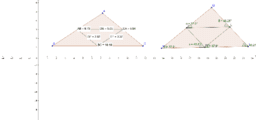# ISG-Dammam Geometry geogebra book

Topic:
Geometry
ISG-Dammam- geometry:Midsegment theorem activity: 1). Create your own worksheet and make two triangles- One to show the relationship between the lengths of the mid-segments of any two sides of a triangle and its third side and the other triangle may be of same side lengths or different lengths does not matter , to show the relationship of the corresponding angles formed by the mid-segments of any two sides of a triangle and its third side. 2). Conjecture the relationship of the lengths of the mid-segments of a triangle. Show it with the help of all 3 sides, try this by dragging the cursor. 3). Conjecture the relationship of the corresponding angles formed by the mid-segments of any two sides of a triangle and its third side. Show this by finding the measures of the corresponding angles formed by the three mid-segments of a triangle and the third side. 4). You could repeat the procedure to make sure with different side lengths of a triangle by dragging the cursor to decide whether your conjecture is true in all cases. 5). What are the two things that you could conjecture from this activity. 6). Does your conjecture match with the mid-segment theorem- Yes/No.